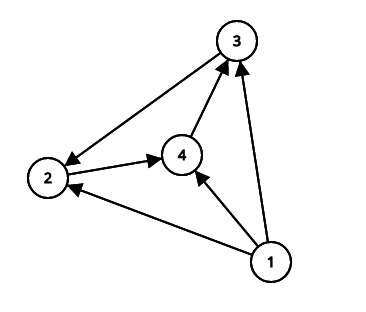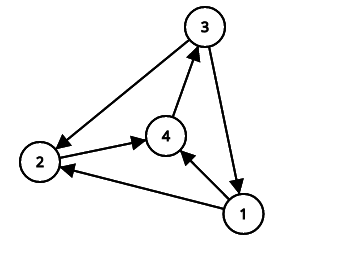Alice and wheel-graph
Tag(s):

## Algorithms, Medium-Hard

Problem
Editorial
Analytics

Alice has a wheel-graph: an undirected graph with $n+1$ nodes and $2 \cdot n$ edges. In wheel-graph there are edges between $n + 1$ and i for each integer $i : 1 \leq i \leq n$ and edges between i and $i\mod n + 1$ for each integer $i : 1 <= i <= n$ (see examples to understand it better). Undirected graphs are quite boring for Alice so she made a directed wheel-graph and now each edge has a direction.

Alice wants performs two types of queries:

• 1 a b - change orientation between nodes a and b. Now orientation is from a to b (before this query in graph was a edge from node b to node a).
• 2 a b - check if is b reachable from a.

Help her.

$INPUT$
The first line of the input contains a single integer n $(2 \leq n \leq 200 000)$ - number of nodes without center node.

Then follow $n \cdot 2$ lines with edges description. The i-th of these lines contains two integers $a_i$ and $b_i$ - index of node the edge start from, index of node the edge goes to. It is guaranteed that edges formed a correct directed wheel-graph with $n + 1$ nodes.

Next line of the input contains a single integer q $(1 \leq q \leq 200 000)$ - number of queries.

Then q lines follow with queries description. The i-th of these lines contains three integers $type_i$, $a_i$, $b_i$ - parameters of the i-th query.

$OUTPUT$
For each query of the second type print "Yes" if b can be reached from a and "No" otherwise.

SAMPLE INPUT
3
1 2
1 3
1 4
2 4
4 3
3 2
5
2 1 2
2 2 1
1 3 1
2 2 1
2 4 1

SAMPLE OUTPUT
Yes
No
Yes
Yes

Explanation

Graph before changeGraph after change in the third queryTime Limit: 3.0 sec(s) for each input file.
Memory Limit: 256 MB
Source Limit: 1024 KB
Marking Scheme: Marks are awarded when all the testcases pass.
Allowed Languages: Bash, C, C++, C++14, Clojure, C#, D, Erlang, F#, Go, Groovy, Haskell, Java, Java 8, JavaScript(Rhino), JavaScript(Node.js), TypeScript, Julia, Kotlin, Lisp, Lisp (SBCL), Lua, Objective-C, OCaml, Octave, Pascal, Perl, PHP, Python, Python 3, R(RScript), Racket, Ruby, Rust, Scala, Swift, Swift-4.1, Visual Basic

## CODE EDITOR

Initializing Code Editor...

## This Problem was Asked inChallenge Name

HackerEarth Collegiate Cup Second Elimination

OTHER PROBLEMS OF THIS CHALLENGE
• Data Structures > Advanced Data Structures
• Math > Linear Algebra
• Math > Number Theory
• Algorithms > Sorting
• Data Structures > Advanced Data Structures
• Data Structures > Advanced Data Structures
• Algorithms > String Algorithms
• Algorithms > Graphs